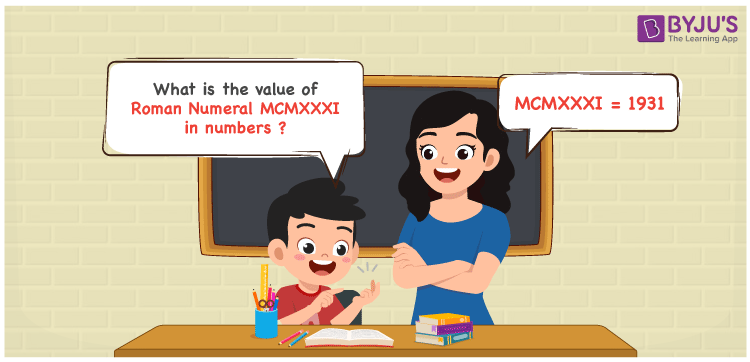Checkout JEE MAINS 2022 Question Paper Analysis : Checkout JEE MAINS 2022 Question Paper Analysis :

# MCMXXXI Roman Numerals

MCMXXXI Roman Numerals is 1931. Roman Numerals are a technique of representing numbers using the Roman alphabet. Students, who get stuck while converting the Roman Numerals into numbers are advised to download the Roman Numerals Conversion in PDF format anytime and erase their doubts instantly. This also improves the learning process among students. Let us understand the method of converting MCMXXXI Roman Numerals into numbers in this article.

 Number Roman Numeral 1931 MCMXXXI

## How to Write MCMXXXI Roman Numerals?The following methods can be used to express the numerical value of MCMXXXI Roman Numerals

First Method:

• Break the Roman Numerals into single letters
• MCMXXXI = M + (M – C) + X + X + X + I
• Write the numerical value of each letter and add/subtract them
• MCMXXXI = 1000 + (1000 – 100) + 10 + 10 + 10 + 1 = 1931

Second Method:

In this method, we consider the groups of Roman Numerals for addition or subtraction

• MCMXXXI = M + CM + XXX + I = 1000 + 900 + 30 + 1 = 1931
• Therefore the numerical value of MCMXXXI Roman Numerals is 1931

## Video Lesson on Roman Numerals## Related Articles

Roman Numerals

Roman Numerals 1 to 50

Roman Numerals 1 to 100

Roman Numerals 1 to 1000

L Roman Numeral

Roman Numeral XXXIX

## Frequently Asked Questions on MCMXXXI Roman Numerals

### What is MCMXXXI Roman Numerals?

MCMXXXI Roman Numerals is 1931.

### Find the sum of MCMXXXI and M Roman Numerals.

MCMXXXI = 1931 and M = 1000. MCMXXXI + M = 1931 + 1000 = 2931. Since 2931 = MMCMXXXI. Hence the sum of MCMXXXI and M Roman Numerals is MMCMXXXI.

### How do you read MCMXXXI Roman Numerals in English?

MCMXXXI Roman Numerals is 1931 and it is read as One Thousand Nine Hundred Thirty-One in English.